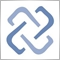Moderator
236222

Regression Channel Indicator and Expert Advisor

Author: boris242406

Hi,

what means:

trailingOn

trailingStart

trailingSize

Meta1[Deleted]

i just wanna know how i can put the RSI, CCI, momentum on this e regr[Deleted]

I have to admit that it looks good. Even when compare iRegr polynomial degree 1 to "fixed" Linear Regression (with " Y = intercept + Slope * X " formula), the shape of Polynomial iRegr is much better. But there's a problem...

The heavy Gauss-matrix calculations is taking too much memory and processor resources, especially when using it as icustom. I end up having MT4 not responding and twice error not enough memory. I tried to reduce the calculation, by having it to calculate several calculation only once, like calculation for sum of square X (which is very easy) or the Gauss-matrix calculation (then we move the matrix result calculation to the second matrix for further calculation, like calculation for polynomial coefficient) and even tried to use different ways for matrix calculation either using Gauss or Gauss-Jordan elimination, or using elementary row calculation to back substitution, but I end up having wrong result value.

My current (and hopefully the only) problem that I can't figure is the Gauss elimination on sum of XY.

I hope its just me the fool one, but is there anyone that can solve this problem, so that the iRegr or another Polynomial Regression doesn't have to calculate on every tick.

The second (little kind a funny) problem is... I rewrite the iRegr so it only draw the first line of the center line and its deviation line only, so I can see the previous lines, but when compare it to LWMA especially on smaller period (<5) the center line looks just almost the same line with LWMA.[Deleted]

I downloaded the EA 2 days ago and installed it (EA) correctly, but it is not trading on GBP/USD-M5. Settings are at default. Any suggestions, please?[Deleted]

Could you pls add a condition such that (True / False) which selects if the EA would initiate trade only in the direction of the trean
Hence

if the Channel is sloping up, only Longs would be initiated and when the proce hits lower band
If the Channel is sloping Down, only Shorts would be initiated when the price hits upper band[Deleted]
onewithzachy:

I have to admit that it looks good. Even when compare iRegr polynomial degree 1 to "fixed" Linear Regression (with " Y = intercept + Slope * X " formula), the shape of Polynomial iRegr is much better. But there's a problem...

The heavy Gauss-matrix calculations is taking too much memory and processor resources, especially when using it as icustom. I end up having MT4 not responding and twice error not enough memory. I tried to reduce the calculation, by having it to calculate several calculation only once, like calculation for sum of square X (which is very easy) or the Gauss-matrix calculation (then we move the matrix result calculation to the second matrix for further calculation, like calculation for polynomial coefficient) and even tried to use different ways for matrix calculation either using Gauss or Gauss-Jordan elimination, or using elementary row calculation to back substitution, but I end up having wrong result value.

My current (and hopefully the only) problem that I can't figure is the Gauss elimination on sum of XY.

I hope its just me the fool one, but is there anyone that can solve this problem, so that the iRegr or another Polynomial Regression doesn't have to calculate on every tick.

The second (little kind a funny) problem is... I rewrite the iRegr so it only draw the first line of the center line and its deviation line only, so I can see the previous lines, but when compare it to LWMA especially on smaller period (<5) the center line looks just almost the same line with LWMA.

Would the following change ensure that the work is done only on the open of each bar?

```int start()
{
if (Bars < bars) return;
if (LastSignal == Time) return;
//----
LastSignal=Time;
```[Deleted]

Great expert, but hard to optimise, has anyone got good optimised inputs ?[Deleted]
Hi, thanks for the EA but I can not run it, install it correctly but no operations. Can you help me? thanks[Deleted]
i-Regr can be modified to apply to a Momentum instead of closing prices ? Merci